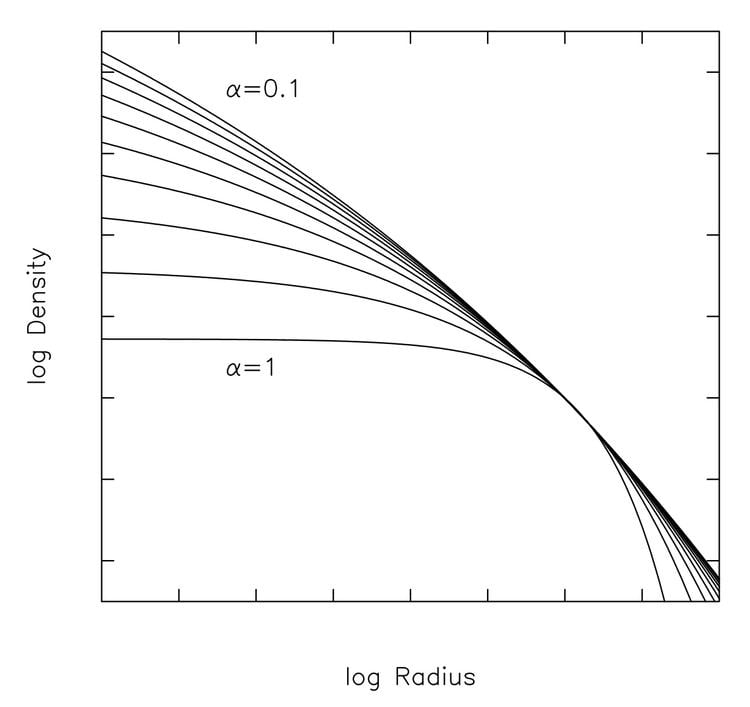# Einasto profile

Updated onThe Einasto profile (or Einasto model) is a mathematical function that describes how the density ρ of a spherical stellar system varies with distance r from its center. Jaan Einasto introduced his model at a 1963 conference in Alma-Ata, Kazakhstan.

The Einasto profile possesses a power law logarithmic slope of the form:

γ ( r ) d ln ρ ( r ) d ln r r α

which can be rearranged to give

ρ ( r ) exp ( A r α ) .

The parameter α controls the degree of curvature of the profile. This can be seen by computing the slope on a log-log plot:

d   ( log ρ ) / d   ( log r ) r α .

The larger α , the more rapidly the slope varies with radius (see figure). Einasto's law can be described as a generalization of a power law, ρ r N , which has a constant slope on a log-log plot.

Einasto's model has the same mathematical form as Sersic's law, which is used to describe the surface brightness (i.e. projected density) profile of galaxies.

Einasto's model has been used to describe many types of system, including galaxies and dark matter halos.

Topics